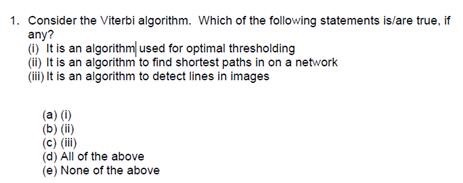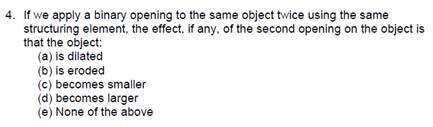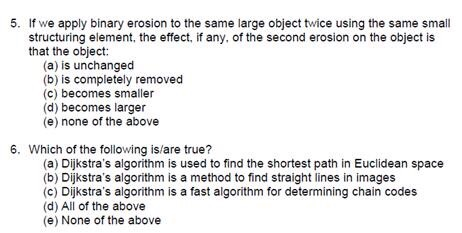1. Consider the Viterbi algorithm. Which of the following statements is/are true. if any? (i) It is an algorithmused for optimal thresholding (ii) It is an algorithm to find shortest paths in on a network (ii)It is an algorithm to detect lines in images (a) (i) (b) (ii) (c) (ii) (d) All of the above (e) None of the above 4. If we apply a binary opening to the same object twice using the same structuring element, the effect. if any. of the second opening on the object is that the object: (a) is dilated (b) is eroded (c) becomes smaller (d) becomes larger (e) None of the above 5. If we apply binary erosion to the same large object twice using the same small structuring element, the effect, if any, of the second erosion on the object is that the object: (a) is unchanged (b) is completely removed (c) becomes smaller (d) becomes larger (e) none of the above 6. Which of the following is/are true? (a) Dijkstra&#39;s algorithm is used to find the shortest path in Euclidean space (b) Dijkstra&#39;s algorithm is a method to find straight lines in images (c) Dijkstra&#39;s algorithm is a fast algorithm for determining chain codes (d) All of the above (e) None of the above